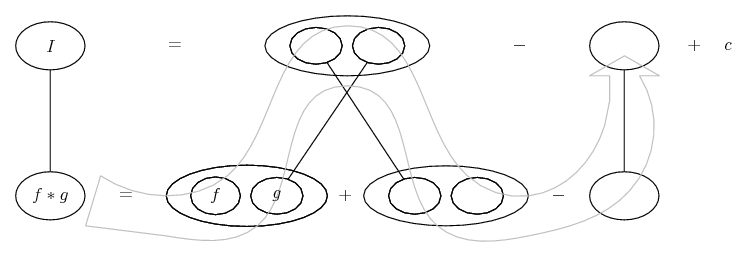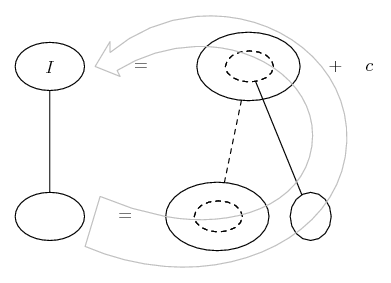# Thread: integration

1. Sorry.

2. 'taking x out' is misleading, but parts maybe wins on simplicity grounds as it circumvents the partial fractions.

Just in case a picture helps...... where (key in spoiler) ...

Spoiler:... is the product rule - straight lines differentiating downwards (integrating up) with respect to x, and...... is lazy integration by parts, doing without u and v.

Spoiler:_________________________________________

Don't integrate - balloontegrate!

Balloon Calculus; standard integrals, derivatives and methods

Balloon Calculus Drawing with LaTeX and Asymptote!

3. just to confirm... is this right?

$\displaystyle \int\frac{x}{(2x+1)^4} dx = -\frac{x}{6(2x+1)^3}$

4. If you look in the bottom spoiler, above, you'll see that what you're offering here as the integral (your RHS) is what I've put at the top of the product rule shape.

But then you can see that differentiating your RHS according to the product rule is going to give you a sum that contains more than the original integrand. I.e. travelling downwards from your alleged integral (minus a sixth of x over the cube of (2x + 1)) you arrive at the original integrand plus some more stuff - in this case, plus (minus a sixth of 1 over the cube of (2x + 1)).

Something like this always happens in integration by parts, but you adjust for it. So on the top row you want to subtract from your alleged integral the integral of the unwanted stuff.

If the pictures aren't helping you to understand integration by parts, someone might do it for you with the usual notation. And bear in mind that this method only arose because sa-ri-ga-ma was indulging your 'taking-x-out' idea (and it turns out to be a good idea, as long as you don't confuse it with 'taking out' a constant); the partial fractions method offered in various of the previous posts is also good.

See also http://www.wolframalpha.com/input/?i...82x%2B1%29%5E4 (click on 'show steps')

Edit: also good, of course, is the substitution suggested originally by sa-ri-ga-ma and now again by Archie Meade, below. You need to learn all these approaches. In particular, you need to see how your method for dealing with one over some power of a linear function is really just a simple special case of the substitution method you apparently 'don't do'. Actually, just in case a picture helps...... where (key in spoiler) ...

Spoiler:... is the chain rule. Straight continuous lines differentiate downwards (integrate up) with respect to the main variable (in this case x), and the straight dashed line similarly but with respect to the dashed balloon expression (the inner function of the composite which is subject to the chain rule).

The general drift is...Spoiler:With partial fractions...Spoiler:So... no, thisOriginally Posted by Punch@tom@ballooncalculus, thanks for yr explanation I assume that what i have posted is right then.. I shall just carry on integrating by removing the numerator if that's the case since it seems easier to understand at least for me
is quite disturbing!
_________________________________________

Don't integrate - balloontegrate!

Balloon Calculus; standard integrals, derivatives and methods

Balloon Calculus Drawing with LaTeX and Asymptote!

5. @tom@ballooncalculus, thanks for yr explanation I assume that what i have posted is right then.. I shall just carry on integrating by removing the numerator if that's the case since it seems easier to understand at least for me

6.Originally Posted by Punchjust to confirm... is this right?

$\displaystyle \int\frac{x}{(2x+1)^4} dx = -\frac{x}{6(2x+1)^3}$
No!

The method you are trying to employ is this....

$\displaystyle \displaystyle\frac{d}{dx}\left(\frac{k}{(2x+1)^3}+ C\right)=k\frac{d}{dx}(2x+1)^{-3}=k(-3)(2x+1)^{-4}(2)=k\frac{-6}{(2x+1)^4}$

$\displaystyle \displaystyle\Rightarrow\int{\frac{-6}{(2x+1)^4}}dx=\frac{1}{(2x+1)^3}+C$

Unfortunately, your "x" in the numerator position is not a constant.
However, using u=2x+1, you get

$\displaystyle u=2x+1\ \Rightarrow\ x=\frac{u-1}{2},\ \frac{du}{2}=dx$

$\displaystyle \displaystyle\int{\frac{x}{(2x+1)^4}}dx=\frac{1}{4 }\left(\int{\frac{u-1}{u^4}}du\right)=\frac{1}{4}\int{\left(\frac{1}{u ^3}-\frac{1}{u^4}\right)du$

for which you can use the technique you were trying

7.Originally Posted by Punchsorry, but i dont integrate with substituting. I learnt another method that is...
i am confused
I shall just carry on integrating by removing the numerator if that's the case since it seems easier to understand at least for me

You need to realize that the process of integration involves many different techniques, not just one technique "that seems easier to understand". There is no single method which works in every situation. The sooner you come to that realization, the sooner you will understand what each responder to this post has been trying to tell you.

#### Search Tags

integration